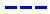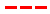Disneyland attraction wait times forecast for June 8, 2019.

•The Average Wait Time We PredictedThe Average Wait Time We Saw
•The Average Wait Time We PredictedThe Average Wait Time We Saw
•The Average Wait Time We PredictedThe Average Wait Time We Saw
•The Average Wait Time We PredictedThe Average Wait Time We Saw
•The Average Wait Time We PredictedThe Average Wait Time We Saw
•The Average Wait Time We PredictedThe Average Wait Time We Saw
•The Average Wait Time We PredictedThe Average Wait Time We Saw
•The Average Wait Time We PredictedThe Average Wait Time We Saw
•The Average Wait Time We PredictedThe Average Wait Time We Saw
•The Average Wait Time We PredictedThe Average Wait Time We Saw
•The Average Wait Time We PredictedThe Average Wait Time We Saw
•The Average Wait Time We PredictedThe Average Wait Time We Saw
•The Average Wait Time We PredictedThe Average Wait Time We Saw
•The Average Wait Time We PredictedThe Average Wait Time We Saw
•The Average Wait Time We PredictedThe Average Wait Time We Saw
•The Average Wait Time We PredictedThe Average Wait Time We Saw
•The Average Wait Time We PredictedThe Average Wait Time We Saw
•The Average Wait Time We PredictedThe Average Wait Time We Saw
•The Average Wait Time We PredictedThe Average Wait Time We Saw
•The Average Wait Time We PredictedThe Average Wait Time We Saw
•The Average Wait Time We PredictedThe Average Wait Time We Saw
•The Average Wait Time We PredictedThe Average Wait Time We Saw
•The Average Wait Time We PredictedThe Average Wait Time We Saw
•The Average Wait Time We PredictedThe Average Wait Time We Saw
•The Average Wait Time We PredictedThe Average Wait Time We Saw
•The Average Wait Time We PredictedThe Average Wait Time We Saw
•The Average Wait Time We PredictedThe Average Wait Time We Saw
•The Average Wait Time We PredictedThe Average Wait Time We Saw
•The Average Wait Time We PredictedThe Average Wait Time We Saw
•The Average Wait Time We PredictedThe Average Wait Time We Saw
•The Average Wait Time We PredictedThe Average Wait Time We Saw
•The Average Wait Time We PredictedThe Average Wait Time We Saw
•The Average Wait Time We PredictedThe Average Wait Time We Saw
•The Average Wait Time We PredictedThe Average Wait Time We Saw
•The Average Wait Time We PredictedThe Average Wait Time We Saw
•The Average Wait Time We PredictedThe Average Wait Time We Saw
•The Average Wait Time We PredictedThe Average Wait Time We Saw
•The Average Wait Time We PredictedThe Average Wait Time We Saw
•The Average Wait Time We PredictedThe Average Wait Time We Saw

The grap